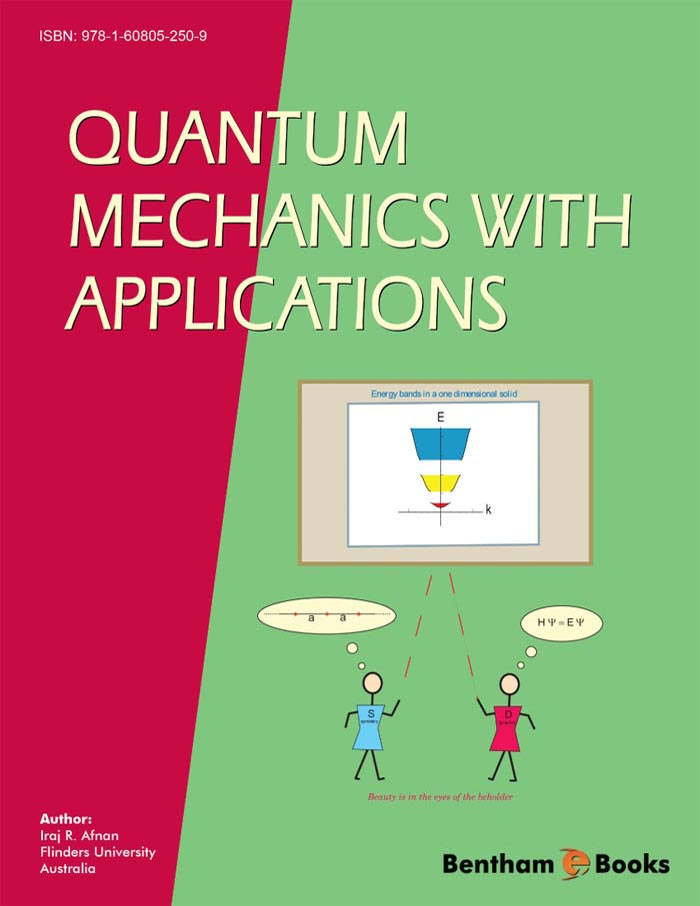Author: Iraj R. Afnan

### Quantum Mechanics with Applications

eBook: US \$44 Special Offer (PDF + Printed Copy): US \$177
Printed Copy: US \$155
ISBN: 978-1-60805-692-7 (Print)
ISBN: 978-1-60805-250-9 (Online)
Year of Publication: 2011
DOI: 10.2174/97816080525091110101

#### Introduction

The ebook introduces undergraduate students to the basic skills required to use non-relativistic quantum mechanics for bound and scattering problems in atomic, molecular and nuclear physics. Initial emphasis is on problems that admit analytic solutions. These results are then used in conjunction with symmetry to develop approximation methods for both bound and scattering problems. The text concentrates on the application of computational problems to introduce the basic concepts of quantum mechanics. These are then used to study more complex problems that can be reduced to one-body problems.

Indexed in: Scopus, EBSCO.

# Preface

The present set of lecture notes are a byproduct of lectures given by the author to undergraduate students at Flinders University over the period of 1970 to 2004. The material in the lecture notes (eBook) was to cover a two semester course. The first semester, at second year level, consisted of two lectures plus one tutorial per week for a period of thirteen weeks, while the second semester, at third year level, was given at the rate of three lectures plus one tutorial per week. The aim of the lectures was to bring the students to a level that would allow them to read the literature in atomic, molecular and nuclear physics and be able to perform some simple calculations based on the non-relativistic quantum mechanics for one- and two-body systems.

With the limited knowledge of calculus, the first semester concentrates on systems in one dimension with extensive applications to atomics, molecules, solids and nuclei. In Chapter 2 we review classical mechanics and the role of space symmetry in conservation laws. Although this chapter is not a prerequisite to later chapters, it gives the students an insight into the relation between classical and quantum physics. The emphasis in the following chapters is to illustrate the mathematical structure of quantum mechanics with examples rather than mathematical proofs. The first semester brings the students to the level of writing the Schrödinger equations for central potentials, e.g. the Coulomb potential.

The second semester of the course commences by considering the solution of the Schrödinger equation as a second order differential equation for simple potentials that admit analytic solutions. This allows us to study the properties of many of the special functions encountered in problems in atomic and nuclear physics. These functions are then used in the application of quantum mechanics to problems in atomic and nuclear physics. The Dirac notation is introduced more as a shorthand notation than as a treatise on Hilbert spaces to understand the importance of the different representations of the Schrödinger equation. Since the symmetry plays a fundamental role in the simplification of problems encountered in quantum physics, a chapter is devoted to space symmetry and the corresponding conservation laws with emphasis on rotational symmetry. As most problems in physics require the introduction of approximation methods, a chapter is devoted to time independent perturbation and variational methods. The last chapter concentrates on the scattering theory based on the Lippmann-Schwinger equation. Here, the solution of the Lippmann-Schwinger equation is illustrated by considering a rank one separable potential for which one can derive an analytic solution for the scattering amplitude, bound state and scattering wave function.

Iraj R. Afnan
Flinders University
Australia